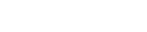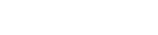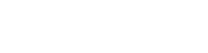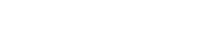## 1.任务CartPole任务的设计使得代理的输入是4个实际值，表示环境状态（位置，速度等）。然而，神经网络可以纯粹通过观察场景来解决任务，因此我们将使用以cart为中心的屏幕补丁作为输入。也因为如此，我们的结果与官方排行榜的结果无法直接比较 – 因为我们的任务要困难得多。而且不幸的是，这确实减慢了训练速度，因为我们必须渲染所有帧。

## 2.需要的包

`torch.nn`
)

`torch.optim`
)

`torch.autograd`

`torchvision`
)- 一个单独的包

```import gym
import math
import random
import numpy as np
import matplotlib
import matplotlib.pyplot as plt
from collections import namedtuple
from itertools import count
from PIL import Image
import torch
import torch.nn as nn
import torch.optim as optim
import torch.nn.functional as F
import torchvision.transforms as T

env = gym.make('CartPole-v0').unwrapped
# set up matplotlib
is_ipython = 'inline' in matplotlib.get_backend()
if is_ipython:
from IPython import display
plt.ion()
# if gpu is to be used
device = torch.device("cuda" if torch.cuda.is_available() else "cpu")```

## 3. 复现记忆（Replay Memory）

`Transition`

`ReplayMemory`

`.sample()`

```Transition = namedtuple('Transition',
('state', 'action', 'next_state', 'reward'))

class ReplayMemory(object):
def __init__(self, capacity):
self.capacity = capacity
self.memory = []
self.position = 0
def push(self, *args):
"""Saves a transition."""
if len(self.memory) < self.capacity:
self.memory.append(None)
self.memory[self.position] = Transition(*args)
self.position = (self.position + 1) % self.capacity
def sample(self, batch_size):
return random.sample(self.memory, batch_size)
def __len__(self):
return len(self.memory)```

## 4. DQN 算法

Q-learning背后的主要思想是，如果我们有一个函数，它可以告诉我们的回报是什幺，如果我们要在给定状态下 采取行动，那幺我们可以轻松地构建最大化我们奖励的政策：## 5. Q_网络（Q_network）

```class DQN(nn.Module):
def __init__(self, h, w, outputs):
super(DQN, self).__init__()
self.conv1 = nn.Conv2d(3, 16, kernel_size=5, stride=2)
self.bn1 = nn.BatchNorm2d(16)
self.conv2 = nn.Conv2d(16, 32, kernel_size=5, stride=2)
self.bn2 = nn.BatchNorm2d(32)
self.conv3 = nn.Conv2d(32, 32, kernel_size=5, stride=2)
self.bn3 = nn.BatchNorm2d(32)
# 线性输入连接的数量取决于conv2d层的输出，因此取决于输入图像的大小，因此请对其进行计算。
def conv2d_size_out(size, kernel_size = 5, stride = 2):
return (size - (kernel_size - 1) - 1) // stride  + 1
convw = conv2d_size_out(conv2d_size_out(conv2d_size_out(w)))
convh = conv2d_size_out(conv2d_size_out(conv2d_size_out(h)))
linear_input_size = convw * convh * 32
# 使用一个元素调用以确定下一个操作，或在优化期间调用batch。返回tensor([[left0exp,right0exp]...]).
def forward(self, x):
x = F.relu(self.bn1(self.conv1(x)))
x = F.relu(self.bn2(self.conv2(x)))
x = F.relu(self.bn3(self.conv3(x)))

## 6. 输入提取

```resize = T.Compose([T.ToPILImage(),
T.Resize(40, interpolation=Image.CUBIC),
T.ToTensor()])

def get_cart_location(screen_width):
world_width = env.x_threshold * 2
scale = screen_width / world_width
return int(env.state * scale + screen_width / 2.0)  # MIDDLE OF CART
def get_screen():
# gym要求的返回屏幕是400x600x3，但有时更大，如800x1200x3。 将其转换为torch order（CHW）。
screen = env.render(mode='rgb_array').transpose((2, 0, 1))
# cart位于下半部分，因此不包括屏幕的顶部和底部
_, screen_height, screen_width = screen.shape
screen = screen[:, int(screen_height*0.4):int(screen_height * 0.8)]
view_width = int(screen_width * 0.6)
cart_location = get_cart_location(screen_width)
if cart_location < view_width // 2:
slice_range = slice(view_width)
elif cart_location > (screen_width - view_width // 2):
slice_range = slice(-view_width, None)
else:
slice_range = slice(cart_location - view_width // 2,
cart_location + view_width // 2)
# 去掉边缘，使得我们有一个以cart为中心的方形图像
screen = screen[:, :, slice_range]
# 转换为float类型，重新缩放，转换为torch张量
# (this doesn't require a copy)
screen = np.ascontiguousarray(screen, dtype=np.float32) / 255
screen = torch.from_numpy(screen)
# 调整大小并添加batch维度（BCHW）
return resize(screen).unsqueeze(0).to(device)

env.reset()
plt.figure()
plt.imshow(get_screen().cpu().squeeze(0).permute(1, 2, 0).numpy(),
interpolation='none')
plt.title('Example extracted screen')
plt.show()```

## 7. 训练

7.1 超参数和实用程序

`select_action`

`EPS_START`

`EPS_END`

`EPS_DECAY`

`plot_durations`

```BATCH_SIZE = 128
GAMMA = 0.999
EPS_START = 0.9
EPS_END = 0.05
EPS_DECAY = 200
TARGET_UPDATE = 10
# 获取屏幕大小，以便我们可以根据AI gym返回的形状正确初始化图层。
# 此时的典型尺寸接近3x40x90
# 这是get_screen（）中的限幅和缩小渲染缓冲区的结果
init_screen = get_screen()
_, _, screen_height, screen_width = init_screen.shape
# 从gym行动空间中获取行动数量
n_actions = env.action_space.n
policy_net = DQN(screen_height, screen_width, n_actions).to(device)
target_net = DQN(screen_height, screen_width, n_actions).to(device)
target_net.eval()
optimizer = optim.RMSprop(policy_net.parameters())
memory = ReplayMemory(10000)

steps_done = 0

def select_action(state):
global steps_done
sample = random.random()
eps_threshold = EPS_END + (EPS_START - EPS_END) * \
math.exp(-1. * steps_done / EPS_DECAY)
steps_done += 1
if sample > eps_threshold:
# t.max(1)将返回每行的最大列值。
# 最大结果的第二列是找到最大元素的索引，因此我们选择具有较大预期奖励的行动。
return policy_net(state).max(1).view(1, 1)
else:

episode_durations = []

def plot_durations():
plt.figure(2)
plt.clf()
durations_t = torch.tensor(episode_durations, dtype=torch.float)
plt.title('Training...')
plt.xlabel('Episode')
plt.ylabel('Duration')
plt.plot(durations_t.numpy())
# 取100个episode的平均值并绘制它们
if len(durations_t) >= 100:
means = durations_t.unfold(0, 100, 1).mean(1).view(-1)
means = torch.cat((torch.zeros(99), means))
plt.plot(means.numpy())
plt.pause(0.001)  # 暂停一下，以便更新图表
if is_ipython:
display.clear_output(wait=True)
display.display(plt.gcf())```

## 8. 训练循环

```def optimize_model():
if len(memory) < BATCH_SIZE:
return
transitions = memory.sample(BATCH_SIZE)
# 转置batch（有关详细说明，请参阅https://stackoverflow.com/a/19343/3343043）。
# 这会将过渡的batch数组转换为batch数组的过渡。
batch = Transition(*zip(*transitions))
# 计算非最终状态的掩码并连接batch元素（最终状态将是模拟结束后的状态）
non_final_mask = torch.tensor(tuple(map(lambda s: s is not None,
batch.next_state)), device=device, dtype=torch.uint8)
non_final_next_states = torch.cat([s for s in batch.next_state
if s is not None])
state_batch = torch.cat(batch.state)
action_batch = torch.cat(batch.action)
reward_batch = torch.cat(batch.reward)
# 计算Q(s_t，a) - 模型计算Q(s_t)，然后我们选择所采取的动作列。
# 这些是根据policy_net对每个batch状态采取的操作
state_action_values = policy_net(state_batch).gather(1, action_batch)
# 计算所有下一个状态的V(s_{t+1})
# non_final_next_states的操作的预期值是基于“较旧的”target_net计算的;
# 用max(1)选择最佳奖励。这是基于掩码合并的，这样我们就可以得到预期的状态值，或者在状态是最终的情况下为0。
next_state_values = torch.zeros(BATCH_SIZE, device=device)
# 计算预期的Q值
expected_state_action_values = (next_state_values * GAMMA) + reward_batch
# 计算Huber损失
loss = F.smooth_l1_loss(state_action_values, expected_state_action_values.unsqueeze(1))
# 优化模型
loss.backward()
for param in policy_net.parameters():
optimizer.step()```

```num_episodes = 50
for i_episode in range(num_episodes):
# 初始化环境和状态
env.reset()
last_screen = get_screen()
current_screen = get_screen()
state = current_screen - last_screen
for t in count():
# 选择动作并执行
action = select_action(state)
_, reward, done, _ = env.step(action.item())
reward = torch.tensor([reward], device=device)
# 观察新的状态
last_screen = current_screen
current_screen = get_screen()
if not done:
next_state = current_screen - last_screen
else:
next_state = None
# 在记忆中存储过渡
memory.push(state, action, next_state, reward)
# 移动到下一个状态
state = next_state
# 执行优化的一个步骤（在目标网络上）
optimize_model()
if done:
episode_durations.append(t + 1)
plot_durations()
break
# 更新目标网络，复制DQN中的所有权重和偏差
if i_episode % TARGET_UPDATE == 0:
print('Complete')
env.render()
env.close()
plt.ioff()
plt.show()```